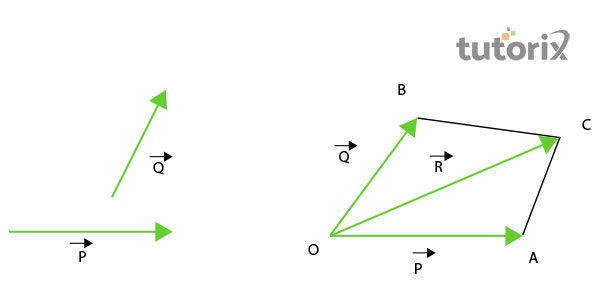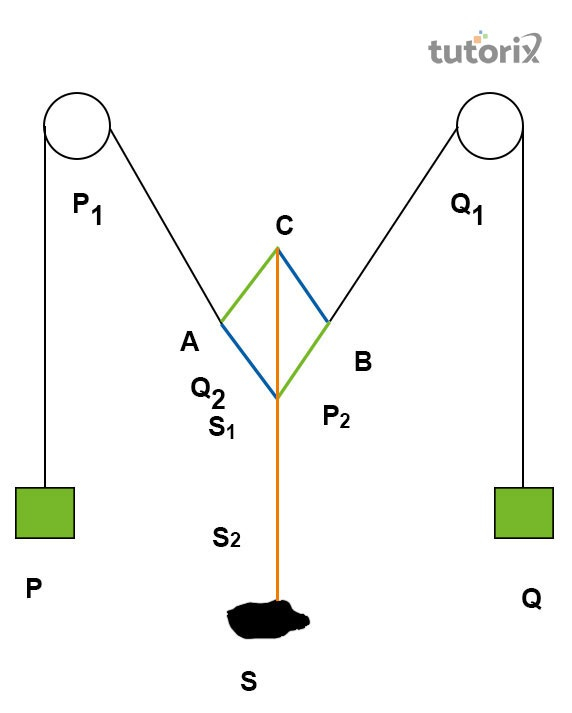# To find the weight of a given Body using Parallelogram Law of Vectors

## Introduction

The use of the vectors law of the Parallelogram is helping in finding the direction of material and it can help develop the notion of an object’s weight. According to the parallelogram law for the addition of the vector, if two vectors can be expressed in extent and direction by the neighbouring sides of a parallelogram, then the consequence is expressed by the parallelogram’s oblique, in both direction and magnitude. This tutorial helps understand if vectors are illustrated in the same direction as slides and the results can appear as the parallelogram’s diagonal. One of the primary purposes of this tutorial is to discover the weight of the given material by involving the law of forces.

## Parallelogram law of vectorsFigure 1: Parallelogram Law of Vectors

A parallelogram is one of the useful principles of diagonal factors that are leading in the measurement purpose of different materials given in a tutorial. The Parallelogram law notes that the aggregate of the squares of the measurement of the four sides of a parallelogram is equivalent to the sum of the squares of the length of the two diagonals. In Euclidean geometry, it is essential that the parallelogram need to include equal opposite sides (Oller, 2019). For instance, if ABCD is a parallelogram is taken for this tutorial, then AB = DC and BC = AD. Hence the definition of the parallelogram will be the same as below: $\mathrm{2(AB)^{2} + 2 (BC)^{2} = (AC)^{2} + (BD)^{2}}$.

## Materials used

In order to fulfil the measurement against the finding weight of any given object according to the Parallelogram law, different materials can be utilised. The following materials have different uses and make the development of the resultant vector. Students who want to make the calculations, need to make appropriate arrangements of the Plumb line, a material whose weight will be measured, two hangers with slotted weights. In the next step, students need to arrange a white paper, scale, strong thread, and pins for drawing (Parween et al. 2018). Additionally, mirror strips and pencils are also required material to complete the measurement.

## Theory

$\mathrm{S\: = \:\sqrt{(P^{2}\: +\: Q^{2} \: +\: 2PQcos\theta)}}$

S is unknown weight; P and Q are the balance weight

## Procedure

In order to determine the weight of a given body through the experiment that is conducted with the help of the Parallelogram Law of Vector, the below-mentioned steps are required to be followed. The placement of the Gravesand's apparatus is required to be on the table in a vertical manner. The main thing that one must need to be sure about is that the pulleys are required to be in a good working order. Between the pulleys a white sheet of paper is required to be secured with the help of thumb pins (Zhang et al. 2021). To the two ends of the thread, the hooks of the hanger weights are required to be tied. The suspension of the unknown weight needs to be ensured by tying another string middle of the string that goes across the pulleys.Figure 2: Diagram of the experiment

A slotted weight will be attached to the hanger and the adjustment of this weight is required until the knot reaches the central part of the sheet. Under each string, a plane mirror is required to be placed for the determination of the directional forces. The end of the mirror strip is required to be marked by placing the eye at6 the place where the string covers the string. After that each pair of the point need to be linked as they can meet at the point O. Then the sides of OA and OB are required to be marked. OCs are measured by joining the marked places diagonally and a spring balance is used in order to be extra sure about the weight of the item. For observing the repetition of the experiment, the weight attached with the P and Q points is needed to be changed for few times.

## Observation and Calculation

Serial Number Forces Sides Resultant force R Unknown weight S Weight by spring balance Error
PQOAOBOC
1.1451453.53.53.61802002055

Table 1: Observation table

The least count of the spring balance and zero error of the spring balance will be determined in the unit of grams (Cohen et al. 2021). The scale that is used is 1centemetr which is equivalent to 50grams. Based on the above observation table, it has been found that OC = 3.6 cm, and R = 50 × 3.6 = 180g. Unknown weight is considered to be equivalent to 200grams whereas the mean unknown weight is calculated as $\mathrm{S\:=\:\frac{S_{1}+S_{2}+S_{3}}{S} \:= \:200g}$. The weight by spring balance is considered similar to the vale 205g and the difference is 5g.

## Conclusion

This tutorial shades light on the factors of the vectors that have potential equation to solve the weight measurement. Most of the time, the given materials are the effective part of the equation where the functional components are relying on the Gravesand's apparatus. Based on the Parallelogram Law of Forces the mentioned materials are useful and require to include the measurement with the functional component and the development of the weight measurement is followed by the procedure that is also furnished above. Additionally, the discussion is made on how to observe the weight of a material by applying the parallelogram law of vectors with the observations.

## FAQs

Q1. What are the precautions one needs to maintain during this experiment?

Ans. The position of the board needs to be stable and vertical and the pulleys must have a low friction coefficient. The middle of the paper required to have the junction O and for the representation of the directions, arrows are required to be used.

Q2. What is a scalar quantity?

Ans. Among the terminology of physics, this particular term, scalar quantity is defined as the physical quality of an object that has only magnitude but direction.